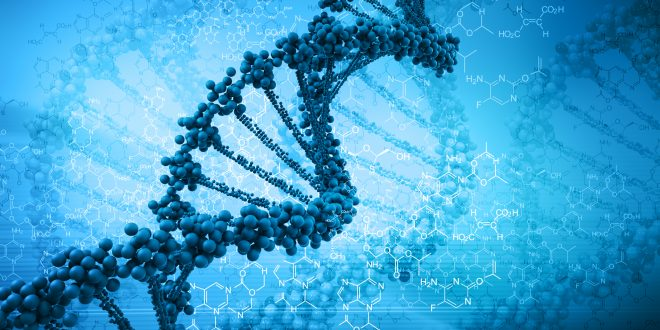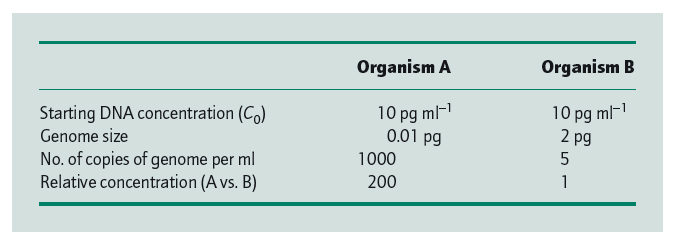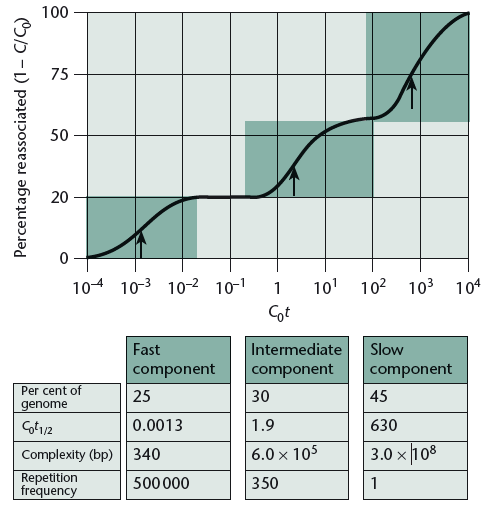https://extremegenes.com/2020/09/06/

# DNA Reassociation Kinetics & Sequence Complexity

When double-stranded DNA in solution is heated, it denatures (‘melts’) releasing the complementary single strands. If the solution is cooled quickly the DNA remains in a single-stranded state. However, if the solution is cooled slowly reassociation/reannealing i. e. spontaneous realignment of two single DNA strands to re-form a DNA double helix will occur. The conditions for efficient DNA re-association were determined originally by Marmur et al. (1963) and since then have been extensively studied by others (for a review, see Tijssen 1993).

To read the previous article for a more basic understanding, click on the text: Genome Size.

The key parameters of reassociation are as follows.

First, there must be an adequate concentration of cations. And below 0.01M sodium ion, there is effectively no reassociation.

Secondly, the temperature of incubation must be high enough to weaken the intrastrand secondary structure. In practice, the optimum temperature for reassociation is 25°C below the melting temperature (Tm), that is, the temperature required to dissociate 50% of the duplex.

Thirdly, the incubation time and the DNA concentration must be sufficient to permit an adequate number of collisions so that the DNA can reassociate.

Finally, the size of the DNA fragments also affects the rate of reassociation and is conveniently controlled if the DNA is sheared into small fragments.

The reassociation of a pair of complementary sequences results from their collision and therefore the rate depends on their concentration. As two strands are involved, the process follows second-order kinetics. Thus, if C is the concentration of DNA that is single-stranded at time t, then

dC/dt = -kC2

or, dC/C2 = -kdt

Here,

• k is the reassociation rate constant.
• dC/dt = Rate of loss of single-stranded DNA during reassociation because reassociation (reannealing) forms double strands from single strands i.e. single strand DNAs are lost with time.

If C0 is the initial concentration of single-stranded DNA at time t = 0, integrating (integration of calculus) the above equation (dC/C2 = -kdt) gives,

C/C0 = 1/(1+kC0t)

Here,

• The unit of C0 is mol/l (mol of nucleotides per liter).
• t is the reassociation time (reannealing time) & its unit is s (second).

Remember,

• If C/C0 = 1, the reassociation is fully complete i.e both the single strands are annealed.
• If C/C0 = 0, no reassociation has taken place.
• If C/C0 = .5, the reassociation is half complete.

When the reassociation is half complete, C/C0 = 0.5, and the above equation simplifies to

C0t1/2 = 1/k

Therefore, t1/2 = 1/k * C0

Here,

• t1/2 = Half period of reassociation.

Thus the greater the C0t1/2 value, the slower the reaction time at a given DNA concentration.

 Fact 1 The greater the C0t1/2 value, the slower the reaction time at a given DNA concentration i.e reassociation will happen slowly.

More important, for a given DNA concentration the half-period (t1/2) for reassociation is proportional to the number of different types of fragments (sequences) present and thus to the genome size (Britten & Kohne 1968). This can best be seen from the data in Table 1. Because the rate of reassociation depends on the concentration of complementary sequences, the C0t1/2 for organism B will be 200 times greater than for organism A.

 Fact 2 The rate of reassociation depends on the relative concentration of complementary sequences.Table 1 Comparison of sequence copy number for two organisms with different genome sizes. Source: Principles of Genome Analysis & Genomics by Primrose & Twyman.

Experimentally it has been shown that the rate of reassociation is indeed dependent on genome size (L = length of the genome) and genome complexity (N). Genome or sequence complexity indicates the presence of repeated sequences in a genome.

• If in a genome or part of a genome, repeated sequences are less, it is less complex.
• If in a genome or part of a genome, repeated sequences are more, it is more complex.
 Fact 3 A sample with a highly-repetitive sequence (less complex) will renature rapidly, while complex sequences will renature slowly.

During reassociation,

a sequence of single-stranded DNA needs to find its complementary strand to reform a double helix, common sequences (less complex genome) renature more rapidly than rare sequences (more complex genome).

With L and N in equations, and as (α is a proportional sign here)

k α (root over L)/N

we get,

C0t1/2 α N/(root over L)

That is,

 Fact 4 If length L is small, C0t1/2 value will be high. If complexity N is small, C0t1/2 value will be low. The opposite will happen if L and N are large.

During this process, the average length of the DNA segment is kept at 200 bases. So, we could think of this as a constant here.

Now, amalgaming facts 1, 3, and 4, we get

 Repetitive DNA will renature at low C0t values, while complex and unique DNA sequences will renature at high C0t values.

So, here we have seen that the C0t1/2 value is actually a measurement of sequence complexity. And the complexity is directly proportional to C0t1/2.

Here we have a graph for you. Suppose, it is of a genome that when reannealed showed three types of reannealing: fast, intermediate, and slow. Here each arrow mark in the graph represents the half period (t1/2) of reassociation or reannealing.The reassociation kinetics of a eukaryotic DNA sample shows the presence of two types of repeated DNA. The arrows indicate the C0t1/2 values for the three components. (Redrawn from Lewin 1994 by permission of Oxford University Press.)

Now, we have some questions for you.

• Explain fact 3 from the table of the graph.
• Explain the curve.

References

• Marmur J., Rownd R. & Schildkraut C.L. (1963) Denaturation and renaturation of deoxyribonucleic acid. Progress in Nucleic Acids Research 1, 231–300.
• Principles of Genome Analysis & Genomics by Primrose & Twyman.
• Tijssen P. (1993) Hybridization with Nucleic Acid Probes. Part 1: Theory and Nucleic Acid Preparation. Elsevier, Amsterdam.
4 1 vote
Article RatingThis is an official account of this website. This ID holds articles from two categories. 1. Articles from our non-authors. 2. Articles of the authors whose IDs were deleted.

## Mutagenic Agents: Types, Mechanism & Irradiation Breeding

Mutation breeding or mutagenesis is one of many great inventions of the 20th century. Until …

Subscribe
Notify of